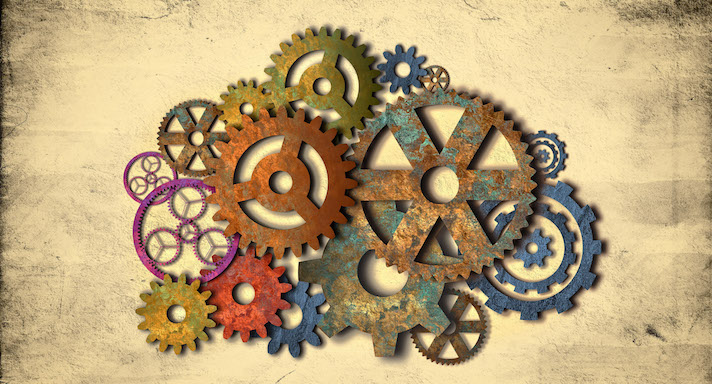# AP Physics### Course Overview

AP Physics 1 is an algebra-based, introductory college-level physics course. Students expand their understanding of Physics through inquiry-based investigations as they explore topics such as:
• Kinematics
• Dynamics
• Circular Motion and Gravitation
• Energy
• Momentum
• Rotational Motion
• Simple Harmonic Motion
• Mechanical Waves
• Electrostatics
• Circuits
• Problem Solving
It is recommended that students complete Honors Physics prior to taking this Algebra-based course. Acellus AP Physics 1 course taught by Greg Spiegel.  This course has been audited and approved by the College Board. Acellus AP Physics is A-G Approved through the University of California.This course was developed by the International Academy of Science. Learn More

### Scope and Sequence

Unit 1 In this unit students review algebra, measuring, and trigonometry. They go on to discuss significant digits, as well as being introduced to vectors.  They learn about describing motion, and about position versus time graphs and velocity versus time graphs.  They experience a virtual graphing lab, and learn about projectile motion. Unit 2 In this unit students learn about force diagrams.  They discuss Newton's First, Second, and Third Laws and experience virtual labs on Newton's Second and Third Laws.  They study friction, forces, inclined planes, and the Atwood Machine. Unit 3 In this unit students study angular measures, circular and angular motion, centripetal force, and circular acceleration.  They explore equations of circular motion, gravity, and Kepler's Laws of Motion.  They further investigate gravitational potential energy and apparent weight. Unit 4  In this unit students learn about work.  They study work and angles, work and inclined planes, kinetic energy, and energy conservation.  They go on to explore mechanical energy and its applications, and to experience a virtual mechanical energy lab.  They finish the unit learning to understand power and efficiency. Unit 5 In this unit students study momentum, impulse, and collisions.  They investigate momentum on a coordinate system and are introduced to impulse, after which they learn to do graphical analysis of impulse.  They further learn about conservation of momentum and about collisions, and experience a virtual conservation of momentum lab. Unit 6 In this unit students come to understand the center of mass, types of motion, rolling motion, and calculations with rolling motion.  They further study torque and changes in rotational motion.  They learn about rotational, static, and mechanical equilibrium, the center of gravity, moment of inertia, and calculations with moment of inertia.  They further investigate rotational work and angular momentum. Unit 7 In this unit students learn about springs and periodic motion as well as springs and mechanical energy.  They experience a virtual lab on springs and periodic motion, and learn about calculations with simple harmonic motion.  They further investigate pendulums, with a virtual, four-part pendulum lab. Unit 8 In this unit students receive a thorough understanding of waves, including transverse and longitudinal waves, waves and phase, sound waves, and frequencies.  They learn about Doppler, wave interference, nodes and antinodes, and wave intensity. Unit 9  In this unit students explore electricity.  They discuss electric charge, net charge and conversion of charge, and conductors and insulators.  They learn about the electroscope, and about charging it with induction and with conduction.  They study the electric field and learn to draw it, and learn about field lines with interacting charges, field lines and conductors, and potential difference. Unit 10  In this unit students begin to understand circuits, starting with drawing circuits, complete circuits, and current.  They learn about resistance and resistivity.  Next they study series circuits.  They learn diagramming series circuits, resistance, voltage, current, and measurements with series circuits, current in a series circuit, and the advantages and disadvantages of the series circuit. Unit 11  Students study parallel circuits in this unit, beginning with diagramming parallel circuits, followed by resistance, voltage, current, measuring current, and measurements in parallel circuits.  They learn the advantages and disadvantages of the parallel circuit, they participate in an experimental analysis of Ohm's Law, and they learn about Kirchhoff. Unit 12  In this unit students review vectors and angles and learn about parabolic motion and reference frame.  They study vertical and horizontal motion, as well as horizontal and vertical components of motion.  They explore projectiles and time aloft, projectiles and launch angle, and motion in the Y-direction.  They receive a thorough understanding of velocity and acceleration. Unit 13 In this unit students come to understand spring constants with both parallel and series springs.  They also learn about pendulum length, angular velocity, and tangential velocity. Unit 14  In this unit, students study applications of Newton's First Law, the Normal Force, gravitational attractions, and centripetal forces and satellites.  They continue to learn about voltage drop and series circuit, inertia and the reference frame, and the Normal Force and inclined planes.  They finish up the course exploring contact forces and tension on a rope.